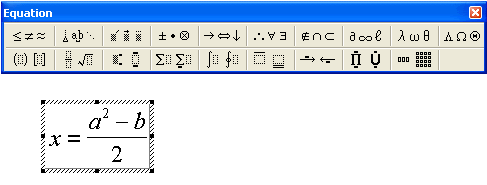# Excel: Using the equation editor

The equation editor is a program that lets you write a mathematical equation into a document, spreadsheet or a slide. Of the programs in the Microsoft Office package, the equation editor is available in Word, Excel and Powerpoint. If you cannot find the equation editor, it may not have been installed on your computer: in this case you must first install it using the installation CD.

## Tabs

Detailed help

You can use the equation editor as follows:

• Choose Object, then Microsoft Equation from the Insert menu.
• Type the equation into the equation frame which appears.• Use the toolbar buttons to pick the correct mathematical operator or form.
• Number values and text can be typed in directly by using the keyboard.
• Move around in the equation as needed by clicking on it with your mouse.
• When the equation is finished, click outside the equation frame.

You can move the equation around and change its size using the mouse. The equation is a so-called embedded object: if you want to edit it, double click the equation.